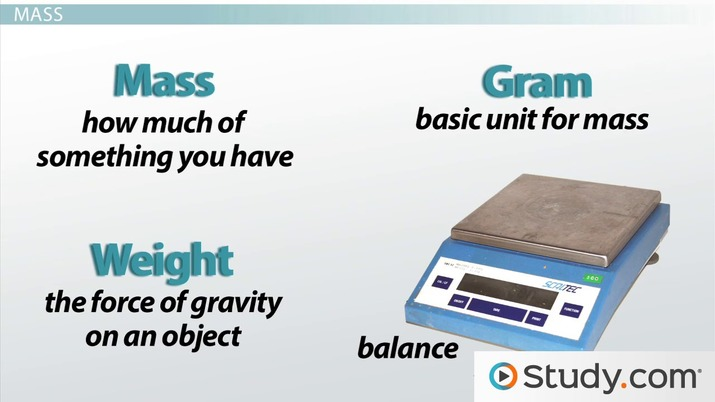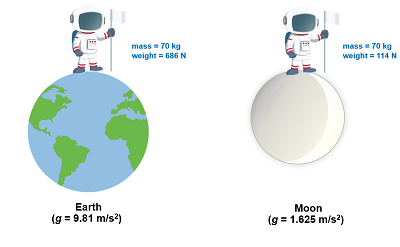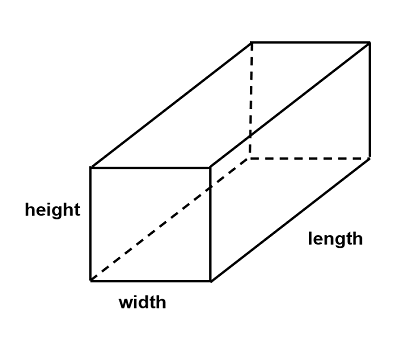# Metric System Units

Rochelle Enrera, Kristin Born, Amanda Robb
• Author
Rochelle Enrera

Rochelle has a bachelor's degree in Physics for Teachers from Philippine Normal University-Manila and has completed 30+ units in MS Geology at University of the Philippines-Diliman. She is a licensed teacher and has taught Grade 10 Physics for three years. She has been a science content writer and copywriter for over three years now.

• Instructor
Kristin Born

Kristin has an M.S. in Chemistry and has taught many at many levels, including introductory and AP Chemistry.

• Expert Contributor
Amanda Robb

Amanda has taught high school science for over 10 years. She has a Master's Degree in Cellular and Molecular Physiology from Tufts Medical School and a Master's of Teaching from Simmons College. She is also certified in secondary special education, biology, and physics in Massachusetts.

Learn about the metric measurements and the metric system units . See how metric system conversion works and compare the measurements to English system. Updated: 11/03/2021

Show

## Metric System: Background

The International System of Units (abbreviated as SI from the French Systeme International d'unites) is the international standard system of measurement. It is commonly known as the metric system, originally developed in France in the late 18th century.

The metric system is based on a factor of 10 or a decimalized system. Prefixes are used to differentiate small from large units. Listed in the table below are the common prefixes used.

Prefix Abbreviation Value Prefix Abbreviation Value
yotta Y 1024 deci d 10-1
zetta Z 1021 centi c 10-2
exa E 1018 milli m 10-3
peta P 1015 micro Greek letter mu 10-6
tera T 1012 nano n 10-9
giga G 109 pico p 10-12
mega M 106 femto f 10-15
kilo k 103 atto a 10-18
hecto h 102 zepto z 10-21
deka da 101 yocto y 10-24

The base 10 system of the metric system explains why it is easier to convert from one unit to another. Its base units are in line with defining constants rather than artifacts and material properties, making it possible to reproduce it anywhere accurately. It has been widely used in science and engineering because the common set of standard units makes it easier for scientists and engineers to replicate and review results from other studies.An error occurred trying to load this video.

Try refreshing the page, or contact customer support.

Coming up next: Unit Conversion and Dimensional Analysis

### You're on a roll. Keep up the good work!

Replay
Your next lesson will play in 10 seconds
• 1:42 Length
• 3:29 Mass
• 4:49 Volume
• 5:32 Density
• 6:58 Temperature
• 8:10 Lesson Summary
Save Save

Want to watch this again later?

Timeline
Autoplay
Autoplay
Speed Speed

## Metric System Units

Different metric system units can be presented along with some common units from the English system of measurement for comparison. The metric system is already standardized and used in most places, while the English system is commonly used in the United States. The metric system only requires using the factors of 10 to convert, while the English system needs a conversion ratio to convert accurately from one unit to another.

### Base Units and Derived Units

Base units comprise all the other units known today. Each base unit is defined by a standard defining constant. What are the base units of the metric system? Refer to the table provided below.

Base Unit Quantity Symbol
second time s
meter length m
kilogram mass kg
ampere electric current A
kelvin thermodynamic temperature K
mole amount of substance mol
candela luminous intensity cd

Derived units are combinations of the base units. For example, area refers to the space occupied by a two-dimensional region. It is a product of two lengths; thus, its unit is m{eq}^2 {/eq}. Other examples of derived units are speed (m/s), density (kg/m{eq}^3 {/eq}), and volume (m{eq}^3 {/eq}).

## Understanding Metric Measurements

Some of the common metric measurements encountered every day are length, mass, volume, density, and temperature. There are different units used to express these quantities.

### Length

Length is a measure of distance. Its SI unit is the meter (m). As a base unit, its technical definition is related to the speed of light in a vacuum at 299 792 458 m/s.

Small distances use prefixes, such as centi- (10{eq}^{-2} {/eq}) and milli- (10{eq}^{-3} {/eq}). In this instance, 1 cm is equal to 0.01 m, and 1 mm is equal to 0.001 m. Common prefixes used for large distances are kilo- (10{eq}^{3} {/eq}) and mega- (10{eq}^{6} {/eq}). A distance of 1 km is equivalent to 1000 m, while 1 Mm is 1 000 000 m.

The basic units of length in the English system are inch, foot, and mile. These units are now defined in terms of meter. One inch (in.) is defined exactly as 2.54 cm, and 1 cm = 0.01 m. Using this definition, one can also easily convert other units to meters. Some of the unit conversions used in the English system are:

• 12 in = 1 ft
• 3 ft = 1 yd
• 5280 ft = 1 mi
• 1760 yd = 1 mi

### Mass

Mass refers to the amount of matter an object contains. Its SI unit equivalent is the kilogram (kg). The previous definition of the kilogram is based on the mass of a platinum-iridium cylinder, but it gradually loses some of its mass, leading to the creation of a more definite and unchanging standard. Today, its definition is tied to the fixed value of the Planck constant at 6.626 070 15 {eq}\times {/eq} 10{eq}^{-34} {/eq} J s.

Among the base units, only the kilogram has a prefix on it. One kilogram is equal to 1000 g. Similar to length, prefixes such as milli- (10{eq}^{-3} {/eq}) for small objects and kilo- (10{eq}^3 {/eq}) for heavier objects express mass in SI units.

The most common units of mass (or weight) in the English system are ounce (oz), pound (lb), and ton. These units are related to the kilogram using the conversion 1 kg {eq}\approx {/eq} 2.205 lb. The conversion ratios of these English units are:

• 16 oz = 1 lb
• 2000 lb = 1 ton

Mass and weight are two different quantities. Mass is the amount of matter contained in an object, while weight measures the amount of gravitational force exerted on an object. Mass remains constant regardless of location, while weight is dependent on an object's location.### Volume

Volume refers to the amount of three-dimensional space occupied or enclosed by an object. It is calculated by multiplying the length, width, and height of an object. It is a product of three lengths, making its derived SI unit as cubic meter or m{eq}^3 {/eq}.Small and large volumes in the metric system are indicated using prefixes. One cubic meter, for example, is equal to 1 000 000 000 mm{eq}^3 {/eq} and 1 000 000 cm{eq}^3 {/eq}. Since these units are not that convenient to use in liquids, the unit liter (L) is used even if it is not an official SI unit. One liter is a special name for a cubic decimeter (dm{eq}^3 {/eq}) and is also equal to 1000 cm{eq}^3 {/eq}. A smaller unit of volume is the milliliter (mL), so 1000 mL = 1 L.

Volumes of solids in the English system are given in cubic feet and cubic yards. The equivalent of these units and other common units of volume of fluids such as fluid ounce (oz), cup (c), pint (pt), quart (qt), gallon (gal) are listed below:

• 1 ft{eq}^3 {/eq} = 1728 in{eq}^3 {/eq}
• 1 yd{eq}^3 {/eq} = 27 ft{eq}^3 {/eq}
• 2 cup = 1 pt
• 32 oz = 1 qt
• 2 pt = 1 qt
• 4 qt = 1 gal

### Density

Density quantifies the degree of compactness of an object and helps determine whether an object will float or sink in water. It is also defined as the mass per unit volume, making it a derived unit. Since the SI unit of mass is kg and the standard unit of volume is m{eq}^3 {/eq}, the SI unit of density is {eq}\frac{kg}{m^3} {/eq}. Other units of density are g/mL and g/cm{eq}^3 {/eq}.

To unlock this lesson you must be a Study.com Member.

• Activities
• FAQs

## Common Conversions

In this activity, students will be practicing measuring different quantities using the metric system and converting between units. For example, students might measure the length of their desk in meters, then convert it to kilometers. Students will choose at least one object for each quantity discussed in the lesson. To do this activity, you'll need a meter stick, a scale to measure mass, and a thermometer that can measure in Celsius. If you only have a ruler or a Fahrenheit thermometer, you can use a simple internet search to help you convert these values into the metric system. Then, let students convert within the metric system on their own.

### Directions

In this activity, you're going to be measuring different objects around you and converting the measurements with the metric system. You should choose one object for each category and initially measure it using the units indicated in the table below. Then, convert it to the other units explained in the lesson. The first row has been filled in as an example. Once you're finished, answer the analysis questions below.

QuantitiesObjectInitial MeasurementConversions
Length (Example)Desk1m0.1km, 100cm, 1000mm
Length
Mass
Volume
Density
Temperature

### Analysis

1. Which quantities were easier to measure and why?
2. Why do you think the metric system is used all over the world?
3. Why do you think that there are different units for each quantity in the metric system, such as meters and kilometers?

### Solutions

Students will probably find length the easiest to measure, since they are all familiar with this linear quantity. Things that students are less familiar with like density or volume might be more difficult. Students should recognize the ease of the metric system, since it is based on a numerical system of tens. Students should also notice that some units are better for measuring certain things. For example, it's easiest to measure a desk in meters, but a larger unit would be needed to measure the distance between cities.

#### What are some examples of metric measurements?

Physical quantities such as length, mass, volume, density, and temperature have corresponding SI units. These are the following: length in m; mass in kg; volume in cubic meter; density in kilogram per cubic meter; and temperature in kelvin. Prefixes (i.e., centi-, centi-, and kilo-) are used to differentiate between small and large quantities.

#### What are the basic metric units?

The seven base units in the metric system (or the SI system) are the meter (m), the kilogram (kg), the second (s), the kelvin (K), the ampere (A), the mole (mol), and the candela (cd).

#### How do you convert metric units to English units?

A conversion factor is used to convert metric units to English units. It is a ratio that shows the relationship between units and is always equal to 1. During conversion, ensure that the unit that needs to be canceled is in the denominator, while the desired unit is placed in the numerator.

### Register to view this lesson

Are you a student or a teacher?